# Precalculus : Descartes' Rule, Intermediate Value Theorem, Sum and Product of Zeros

## Example Questions

### Example Question #1 : Find The Sum And Product Of The Zeros Of A Polynomial

Given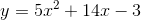, determine the sum and product of the zeros respectively.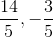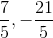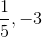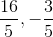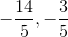Explanation:

To determine zeros of, factorize the polynomial.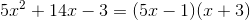Set each of the factorized components equal to zero and solve for.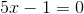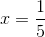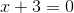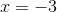The sum of the roots: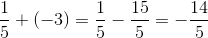The product of the roots: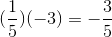### Example Question #2 : Find The Sum And Product Of The Zeros Of A Polynomial

Find the sum and product of the zeros of the following polynomial: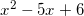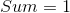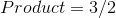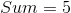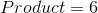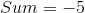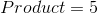Explanation:

To find the zeros you have to factor the polynomial.

This is easily factorable and you will get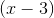and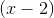.

Next, set both of these equal to zero.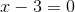and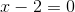.

Isolate the x's and you will getand.

The sum will besince you add the two together, and the product will bebecause you multiply the two together.

### Example Question #1 : Find The Sum And Product Of The Zeros Of A Polynomial

Find the sum and product of the zeros of the following polynomial: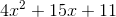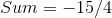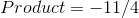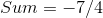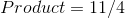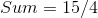Explanation:

Factoring the polynomial will give you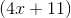and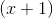.

The zeros of these binomials will be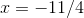and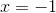.

If you add these together (sum) you get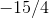and if you multiply them together (product) you get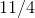.

### Example Question #4 : Descartes' Rule, Intermediate Value Theorem, Sum And Product Of Zeros

Determine the possible number of Positive and Negative Real Zeros of the polynomial using Descartes' Rule of Signs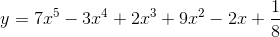Positive zeroes: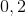Negative zeroes: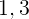Positive zeroes:Negative zeroes:Positive zeroes:Negative zeroes:Positive zeroes: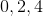Negative zeroes:Positive zeroes:Negative zeroes:Explanation:

In order to determine the positive number of real zeroes, we must count the number of sign changes in the coefficients of the terms of the polynomial. The number of real zeroes can then be any positive difference of that number and a positive multiple of two.

For the functionthere are four sign changes. As such, the number of positive real zeroes can beIn order to determine the positive number of real zeroes, we must count the number of sign changes in the coefficients of the terms of the polynomial after substituting for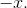The number of real zeroes can then be any positive difference of that number and a positive multiple of two.

After substituting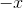we get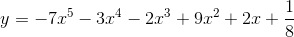and there is one sign change.

As such, there can only be one negative root.

### All Precalculus Resources